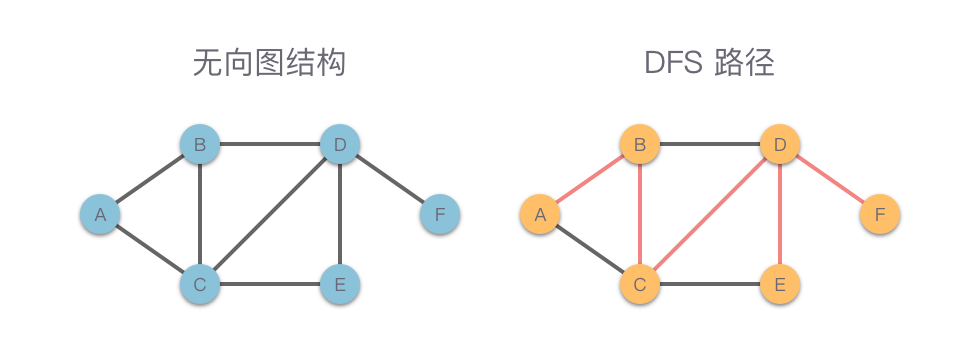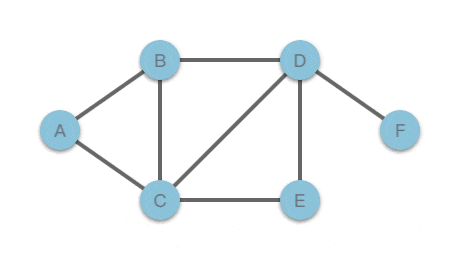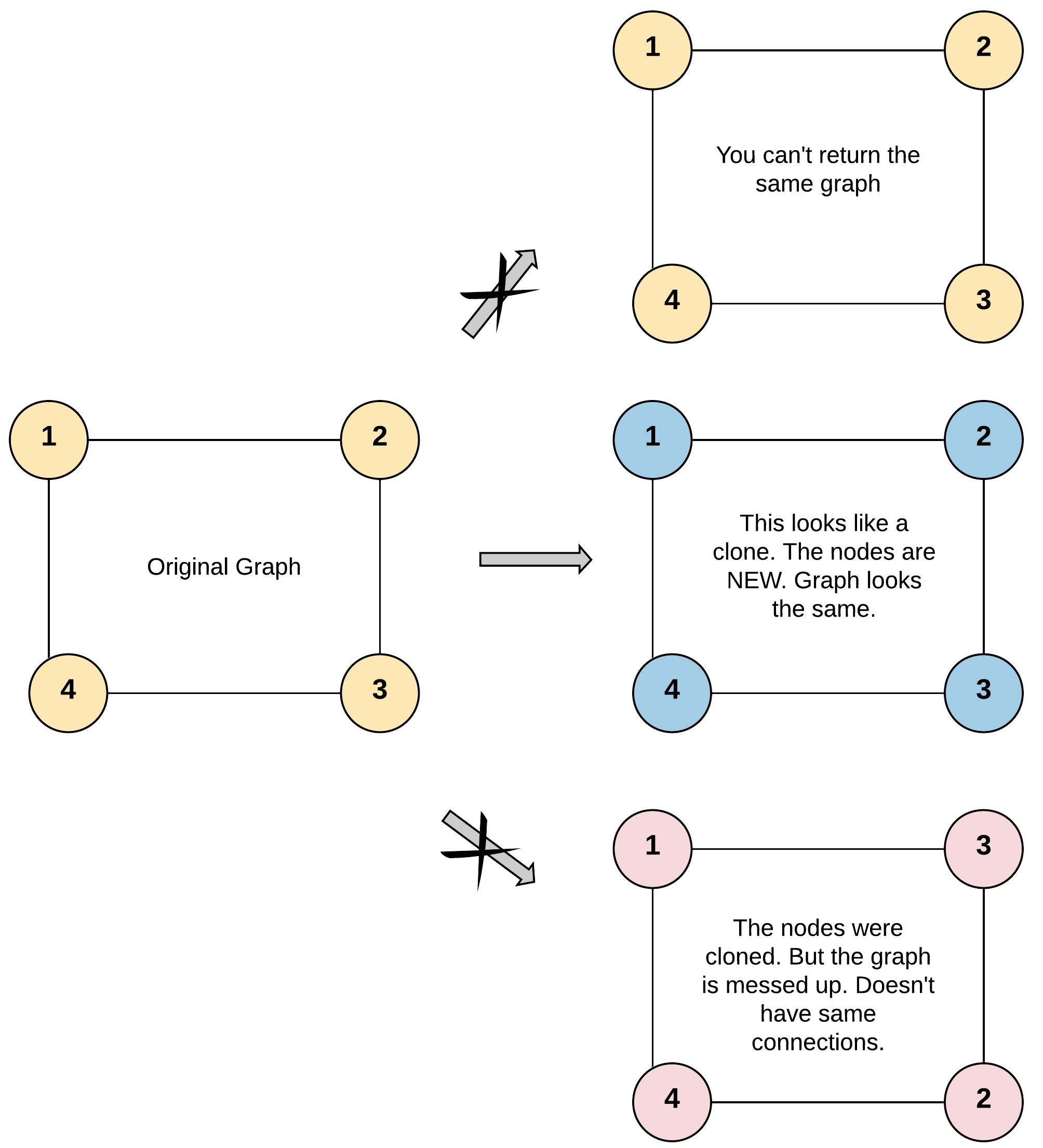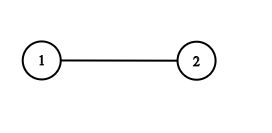01.图的深度优先搜索知识

# 图的深度优先搜索知识 #

## 2. 深度优先搜索过程演示 #

 1 2 3 4 5 6 7 8 9  # 定义无向图结构 graph = { "A": ["B", "C"], "B": ["A", "C", "D"], "C": ["A", "B", "D", "E"], "D": ["B", "C", "E", "F"], "E": ["C", "D"], "F": ["D"] }## 3. 基于递归实现的深度优先搜索 #

### 3.1 基于递归实现的深度优先搜索实现步骤 #

1. 定义 graph 为存储无向图的字典变量，visited 为标记访问节点的 set 集合变量。start 为当前遍历边的开始节点。def dfs_recursive(graph, start, visited): 为递归实现的深度优先搜索方法。
2. start 标记为已访问，即将 start 节点放入 visited 中（visited.add(start)）。
3. 访问节点 start，并对节点进行相关操作（看具体题目要求）。
4. 遍历与节点 start 相连并构成边的节点 end
1. 如果 end 没有被访问过，则从 end 节点调用递归实现的深度优先搜索方法，即 dfs_recursive(graph, end, visited)

### 3.2 基于递归实现的深度优先搜索实现代码 #

  1 2 3 4 5 6 7 8 9 10  def dfs_recursive(graph, start, visited): # 标记节点 visited.add(start) # 访问节点 print(start) for end in graph[start]: if end not in visited: # 深度优先遍历节点 dfs_recursive(graph, end, visited) 

## 4. 基于堆栈实现的深度优先搜索 #

### 4.1 基于堆栈实现的深度优先搜索实现步骤 #

1. start 为开始节点。定义 visited 为标记访问节点的 set 集合变量。定义 stack 用于存放临时节点的栈结构。
2. 首先访问起始节点，并对节点进行相关操作（看具体题目要求）。
3. 然后将起始节点放入栈中，并标记访问。即 visited = set(start)stack = [start]
4. 如果栈不为空，取 stack 栈顶元素 node_u
5. 遍历与节点 node_u 相连并构成边的节点 node_v
• 如果 node_v 没有被访问过，则：
• 访问节点 node_v，并对节点进行相关操作（看具体题目要求）。
• node_v 节点放入栈中，并标记访问，即 stack.append(node_v)visited.add(node_v)
• 跳出遍历 node_v 的循环。
• 继续遍历 node_v
6. 如果 node_u 相邻的节点都访问结束了，从栈顶弹出 node_u，即 stack.pop()
7. 重复步骤 4 ~ 6，直到 stack 为空。

### 4.2 基于堆栈实现的深度优先搜索实现代码 #

  1 2 3 4 5 6 7 8 9 10 11 12 13 14 15 16 17 18 19 20 21  def dfs_stack(graph, start): print(start) # 访问节点 start visited = set(start) # 使用 visited 标记访问过的节点，先标记 start stack = [start] # 创建一个栈，并将 start 加入栈中 while stack: node_u = stack[-1] # 取栈顶元素 i = 0 while i < len(graph[node_u]): # 遍历栈顶元素，遇到未访问节点，访问节点并跳出。 node_v = graph[node_u][i] if node_v not in visited: # node_v 未访问过 print(node_v) # 访问节点 node_v stack.append(node_v) # 将 node_v 加入栈中 visited.add(node_v) # 标记为访问过 node_v break i += 1 if i == len(graph[node_u]): # node_u 相邻的节点都访问结束了，弹出 node_u stack.pop() 

## 5. 深度优先搜索应用 #

### 5.1 岛屿数量 #

#### 5.1.2 题目大意 #

• 岛屿总是被水包围，并且每座岛屿只能由水平方向和/或竖直方向上相邻的陆地连接形成。
• 此外，你可以假设该网格的四条边均被水包围。
• $m == grid.length$。
• $n == grid[i].length$。
• $1 \le m, n \le 300$。
• grid[i][j] 的值为 '0''1'

  1 2 3 4 5 6 7 8 9 10 11 12 13 14 15 16  输入：grid = [ ["1","1","1","1","0"], ["1","1","0","1","0"], ["1","1","0","0","0"], ["0","0","0","0","0"] ] 输出：1 输入：grid = [ ["1","1","0","0","0"], ["1","1","0","0","0"], ["0","0","1","0","0"], ["0","0","0","1","1"] ] 输出：3 

#### 5.1.3 解题思路 #

##### 思路 1：深度优先搜索 #
1. 遍历 grid
2. 对于每一个字符为 '1' 的元素，遍历其上下左右四个方向，并将该字符置为 0，保证下次不会被重复遍历。
3. 如果超出边界，则返回 0
4. 对于 (i, j) 位置的元素来说，递归遍历的位置就是 (i - 1, j)(i, j - 1)(i + 1, j)(i, j + 1) 四个方向。每次遍历到底，统计数记录一次。
5. 最终统计出深度优先搜索的次数就是我们要求的岛屿数量。
##### 思路 1：代码 #
  1 2 3 4 5 6 7 8 9 10 11 12 13 14 15 16 17 18 19 20  class Solution: def dfs(self, grid, i, j): n = len(grid) m = len(grid) if i < 0 or i >= n or j < 0 or j >= m or grid[i][j] == '0': return 0 grid[i][j] = '0' self.dfs(grid, i + 1, j) self.dfs(grid, i, j + 1) self.dfs(grid, i - 1, j) self.dfs(grid, i, j - 1) def numIslands(self, grid: List[List[str]]) -> int: count = 0 for i in range(len(grid)): for j in range(len(grid)): if grid[i][j] == '1': self.dfs(grid, i, j) count += 1 return count 
##### 思路 1：复杂度分析 #
• 时间复杂度：$O(m \times n)$。其中 $m$ 和 $n$ 分别为行数和列数。
• 空间复杂度：$O(m \times n)$。

### 5.2 克隆图 #

#### 5.2.2 题目大意 #

• 节点数不超过 100
• 每个节点值 $Node.val$ 都是唯一的，$1 \le Node.val \le 100$。
• 无向图是一个简单图，这意味着图中没有重复的边，也没有自环。
• 由于图是无向的，如果节点 p 是节点 q 的邻居，那么节点 q 也必须是节点 p 的邻居。
• 图是连通图，你可以从给定节点访问到所有节点。1 2 3 4 5 6 7 8  输入：adjList = [[2,4],[1,3],[2,4],[1,3]] 输出：[[2,4],[1,3],[2,4],[1,3]] 解释： 图中有 4 个节点。 节点 1 的值是 1，它有两个邻居：节点 2 和 4 。 节点 2 的值是 2，它有两个邻居：节点 1 和 3 。 节点 3 的值是 3，它有两个邻居：节点 2 和 4 。 节点 4 的值是 4，它有两个邻居：节点 1 和 3 。1 2  输入：adjList = [,] 输出：[,] 

#### 5.2.3 解题思路 #

##### 思路 1：深度优先搜索 #
1. 使用哈希表 visitedDict 来存储原图中被访问过的节点和克隆图中对应节点，键值对为 原图被访问过的节点：克隆图中对应节点。
2. 从给定节点开始，以深度优先搜索的方式遍历原图。
1. 如果当前节点被访问过，则返回隆图中对应节点。
2. 如果当前节点没有被访问过，则创建一个新的节点，并保存在哈希表中。
3. 遍历当前节点的邻接节点列表，递归调用当前节点的邻接节点，并将其放入克隆图中对应节点。
3. 递归结束，返回克隆节点。
##### 思路 1：代码 #
  1 2 3 4 5 6 7 8 9 10 11 12 13 14 15 16 17  class Solution: def cloneGraph(self, node: 'Node') -> 'Node': if not node: return node visitedDict = dict() def dfs(node: 'Node') -> 'Node': if node in visitedDict: return visitedDict[node] clone_node = Node(node.val, []) visitedDict[node] = clone_node for neighbor in node.neighbors: clone_node.neighbors.append(dfs(neighbor)) return clone_node return dfs(node) 
##### 思路 1：复杂度分析 #
• 时间复杂度：$O(n)$。其中 $n$ 为图中节点数量。
• 空间复杂度：$O(n)$。